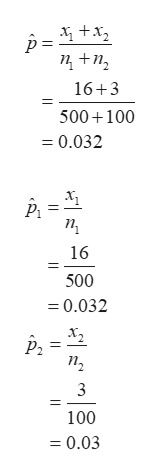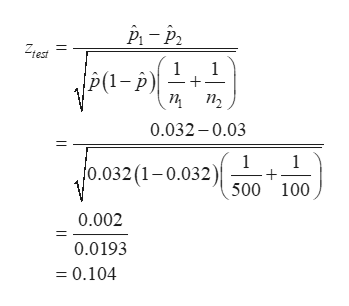# A machine puts out 16 imperfect articles in a sample of 500. After the machine is overhauled it puts 3 imperfect articles in a batch of 100. Has the machine been improved? Use 5% level of significance. Use p-value fot your conclusion and also find 88% confidence interval for difference between population proportion.

Question
143 views

A machine puts out 16 imperfect articles in a sample of 500. After the machine is overhauled it puts 3 imperfect articles in a batch of 100. Has the machine been improved? Use 5% level of significance. Use p-value fot your conclusion and also find 88% confidence interval for difference between population proportion.

check_circle

Step 1

From the given information, the claim of the problem is the machine has been improved.

Null Hypothesis:

H0:p1=p2

Alternative Hypothesis:

Ha:p1-p2<0

Test Statistic:

From the given information, n1=500, x1=16, n2=100 and x2=3.

The pooled sample proportion is,help_outlineImage TranscriptioncloseX, +x, п+п, 16+3 500+100 = 0.032 п, 16 500 = 0.032 X2 п, 3 100 = 0.03 || || fullscreen
Step 2

The test statistic is 0.104 and it is calculated below:help_outlineImage TranscriptioncloseZtest a- (1– P) п п, 0.032 – 0.03 (0.032 (1-0.032) 500 100 0.002 0.0193 = 0.104 || fullscreen
Step 3

p-value:

The p-value is 0.5414 and it is calculated below:

...help_outlineImage Transcriptionclosep-value = P(z < Ziest ) [Since, the hypothesis is left tail test] = P(z <0.104) [=NORM.DIST(0.104,0,1,TRUE)] =0.5414 fullscreen

### Want to see the full answer?

See Solution

#### Want to see this answer and more?

Solutions are written by subject experts who are available 24/7. Questions are typically answered within 1 hour.*

See Solution
*Response times may vary by subject and question.
Tagged in

### Probability